# Conditional Format Dates earlier than or Greater Than Today

This post will guide you how to use conditional formatting to identify dates earlier than or greater than today in Excel. How do I highlight dates greater than or less than today or a certain date with conditional formatting in Excel. How to format cell if cell contains date less than or greater than today in Excel.

## Conditional Format Dates earlier than or Greater Than Today

Assuming that you have a list of data in range B1:B6 which contain dates, and you want to format dates that are older than the current date or greater than today. How to achieve it. You can use the conditional formatting feature to achieve the result. Just do the following steps:

#1 select the data that contain dates.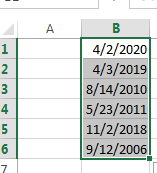#2 go to HOME tab, click Conditional Formatting command under Styles group, and select New Rules from the popup menu list. And the New Formatting Rule dialog will open.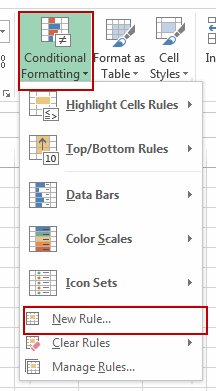#3 select Use a formula to determine which cells to format in the Select a Rule Type section. Then type the following formula into the Format values where this formula is true text box.

=\$B1 >TODAY() (greater than today)

Or

=\$B1 < TODAY() (earlier than today)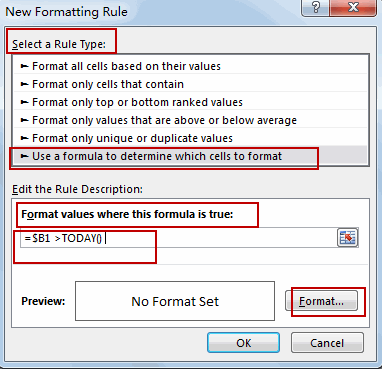#4 click Format button and the Format Cells dialog will open. You can set the formats for dates, such as: fonts or background color. Click OK button.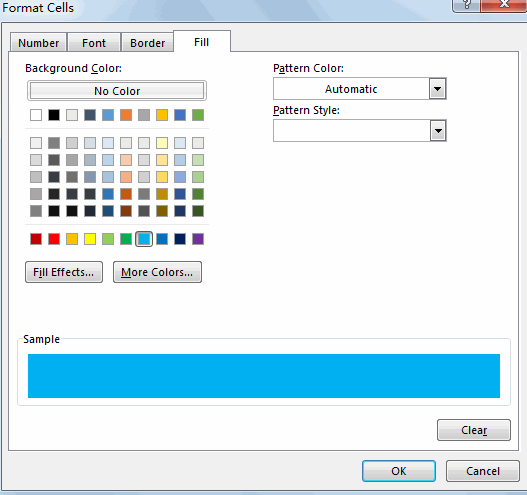#5 click Ok button.

All dates greater than or earlier than today are formatted.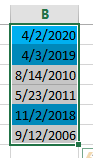Related Functions

• Excel TODAY function
The Excel TODAY function returns the serial number of the current date. So you can get the current system date from the TODAY function. The syntax of the TODAY function is as below:=TODAY()…
Related Posts

Working Time Calculation Based on Timesheets

In the office, a special machine record the time when you start working (clock in office) and when you finish working (clock out of office). We can calculate the total working time by subtracting the end working time from the ...

Basic Price Discount Calculation with Excel VLOOKUP Function

We often encounter product discounts in our shopping. Depending on the level of spending, the mall will offer different percentages of discounts. Usually, the more you spend, the bigger the discount, while the less you spend, the smaller the discount. ...

Sort/Rank Numeric Values with Duplicate Values Exist

Excel built-in RANK function can sort a set of values. If there are duplicate numbers, then the rank number is also duplicated. See the following example: There are two numbers “100” in range A2:A9, they are both the third largest ...

Calculate Days Open in Excel

If you want to know how to Calculate days in Excel, there are some formulas that you can use to do so. For example, you can use the DAYS function in Excel to find the number of days between two ...

Calculate Grades With VLookup in Excel

Why Should You Calculate Grades With VLookup Excel? If you're looking for a simple way to find out a student's grades, VLOOKUP Excel can do the trick. This function uses a lookup table to find the values and sort them ...

How to Sum Last N Days in Excel

In our daily life, we may want to sum amounts or sales for a specific period, for example in last N days. Sum numbers in Excel is easy to run, we can apply SUM function. But if we want to ...

How to Calculate Retirement Date and Remaining Years refer to Birth Date in Excel

Every company has its own police about the retirement date for employees. Suppose employees will be retired from a certain age of 60, how can we calculate the retirement date for everyone base on their birthdays? Actually, there are several ...

How to Sum If Date is Greater or Less Than in Excel

If we sum for certain conditions, we'll use the SUMIF function in excel. For example, count the total sales for a period before of after a specified date. This article will help you to do sum for a specified period ...

How to Highlight Cell or Row If Date Is In Current Day/Week/Month in Excel

This post will guide you how to highlight cell if date is the current day or is in the current week or month in Excel. How do I highlight row if date is in current week or month with conditional ...

How to Add the Current Month or Year in a Cell in Excel

This post will guide you how to add the current month or year into a cell or header or footer in your worksheet. How do I insert the current date or time in a cell with a formula in Excel. ...

Sidebar